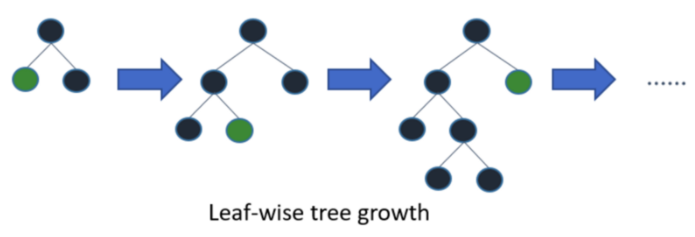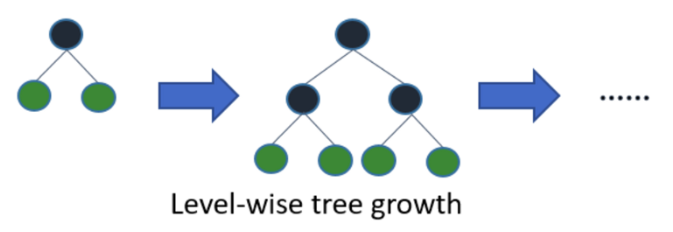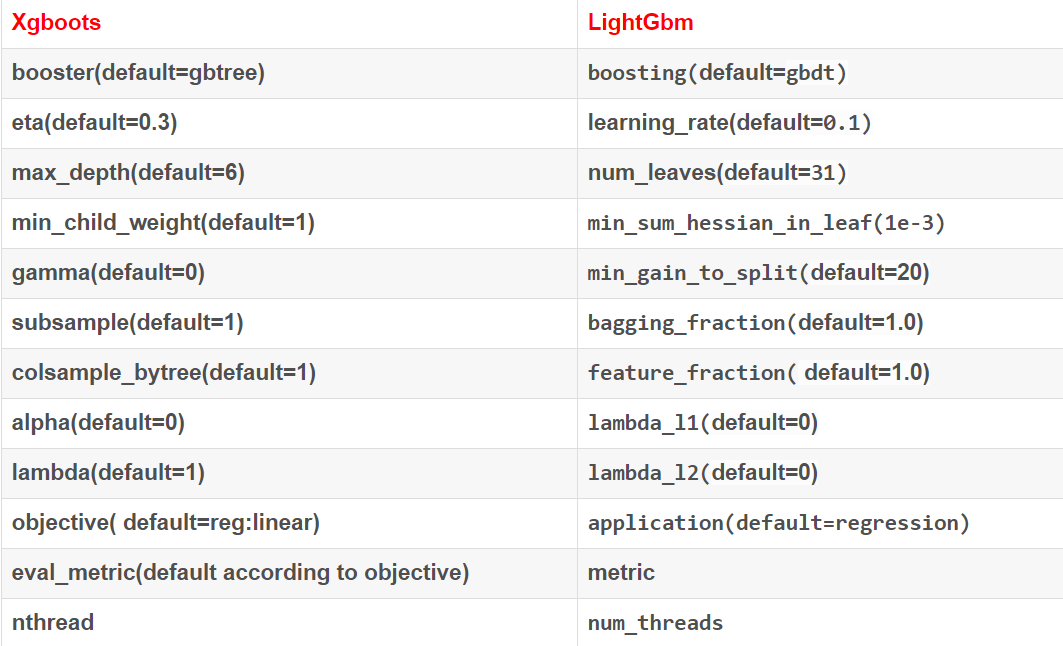# 1.简介

lightGBM包含两个关键点：light即轻量级，GBM 梯度提升机

LightGBM 是一个梯度 boosting 框架，使用基于学习算法的决策树。它可以说是分布式的，高效的，有以下优势：

• 更快的训练效率

• 低内存使用

• 更高的准确率

• 支持并行化学习

• 可处理大规模数据

LightGBM 垂直地生长树，即 leaf-wise，它会选择最大 delta loss 的叶子来增长。# 2.特点

## 1）xgboost缺点

• 每轮迭代时，都需要遍历整个训练数据多次。如果把整个训练数据装进内存则会限制训练数据的大小；如果不装进内存，反复地读写训练数据又会消耗非常大的时间。

• 预排序方法（pre-sorted）：首先，空间消耗大。这样的算法需要保存数据的特征值，还保存了特征排序的结果（例如排序后的索引，为了后续快速的计算分割点），这里需要消耗训练数据两倍的内存。其次时间上也有较大的开销，在遍历每一个分割点的时候，都需要进行分裂增益的计算，消耗的代价大。

• 对cache优化不友好。在预排序后，特征对梯度的访问是一种随机访问，并且不同的特征访问的顺序不一样，无法对cache进行优化。同时，在每一层长树的时候，需要随机访问一个行索引到叶子索引的数组，并且不同特征访问的顺序也不一样，也会造成较大的cache miss。

## 2）LightGBM优点

Histogram算法

Level-wise过一次数据可以同时分裂同一层的叶子，容易进行多线程优化，也好控制模型复杂度，不容易过拟合。但实际上Level-wise是一种低效算法，因为它不加区分的对待同一层的叶子，带来了很多没必要的开销，因为实际上很多叶子的分裂增益较低，没必要进行搜索和分裂。

Leaf-wise则是一种更为高效的策略：每次从当前所有叶子中，找到分裂增益最大的一个叶子，然后分裂，如此循环。因此同Level-wise相比，在分裂次数相同的情况下，Leaf-wise可以降低更多的误差，得到更好的精度。

Leaf-wise的缺点：可能会长出比较深的决策树，产生过拟合。因此LightGBM在Leaf-wise之上增加了一个最大深度限制，在保证高效率的同时防止过拟合。

# 3.安装

pip install lightgbm

# 4.参数

• max_depth, default=-1, type=int，树的最大深度限制，防止过拟合
• min_data_in_leaf, default=20, type=int, 叶子节点最小样本数，防止过拟合
• feature_fraction, default=1.0, type=double, 0.0 < feature_fraction < 1.0,随机选择特征比例，加速训练及防止过拟合
• feature_fraction_seed, default=2, type=int，随机种子数，保证每次能够随机选择样本的一致性
• bagging_fraction, default=1.0, type=double, 类似随机森林，每次不重采样选取数据
• lambda_l1, default=0, type=double, L1正则
• lambda_l2, default=0, type=double, L2正则
• min_split_gain, default=0, type=double, 最小切分的信息增益值
• top_rate, default=0.2, type=double，大梯度树的保留比例
• other_rate, default=0.1, type=int，小梯度树的保留比例
• min_data_per_group, default=100, type=int，每个分类组的最小数据量
• max_cat_threshold, default=32, type=int，分类特征的最大阈值
• num_leaves：默认 31，因为LightGBM使用的是leaf-wise的算法，因此在调节树的复杂程度时，使用的是num_leaves而不是max_depth。
大致换算关系：num_leaves = 2^(max_depth)。它的值的设置应该小于2^(max_depth)，否则可能会导致过拟合。
• 对于非平衡数据集：可以param['is_unbalance']='true’
• early_stopping_round：如果一次验证数据的一个度量在最近的early_stopping_round 回合中没有提高，模型将停止训练，加速分析，减少过多迭代
• application（objective）：选择 regression: 回归时，binary: 二分类时，multiclass: 多分类时
• num_class：多分类的分类数
• learning_rate：如果一次验证数据的一个度量在最近的 early_stopping_round 回合中没有提高，模型将停止训练，常用 0.1, 0.001, 0.003…
• verbose_eval：type=int，输出日志详细情况，多少条数据输出一条记录

# 5.比较# 6.调参

Faster Speedbetter accuracyover-fitting
max_bin 设置小一些 用较大的 max_bin max_bin 小一些
num_leaves 大一些 num_leaves 小一些
feature_fraction 来做 sub-sampling   feature_fraction
bagging_fraction 和 bagging_freq   设定 bagging_fraction 和 bagging_freq
training data 多一些 training data 多一些
save_binary 来加速数据加载 直接用 categorical feature gmin_data_in_leaf 和 min_sum_hessian_in_leaf

num_iterations 大一些，learning_rate 小一些 max_depth 控制树的深度

# 7.例子

params={
"learning_rate":0.1,
"lambda_l1":0.1,
"lambda_l2":0.2,
'num_leaves':20,
"max_depth":4,
"objective":"multiclass",
"num_class":11,
}

param['is_unbalance']='true'
param['metric'] = 'auc'

clf=lgb.train(params,train_data,num_boost_round=100000,early_stopping_rounds=50,verbose_eval=20)
pred = clf.predict(X_test,num_iteration=clf.best_iteration)

# 8.自动寻优调参

import lightgbm as lgb
import pandas as pd
from sklearn.metrics import mean_squared_error
from sklearn.model_selection import GridSearchCV

estimator = lgb.LGBMRegressor(objective='regression',colsample_bytree=0.8,subsample=0.9,subsample_freq=5)

param_grid={
'learning_rate':[0.01,0.02,0.05,0.1],
'n_estimators' :[1000,2000,3000,4000,5000],
'num_leaves':[128,1024,4096]
}

fit_param={'categorical_feature':[0,1,2,3,4,5]}
gbm = GridSearchCV(estimator,param_grid,fit_params=fit_param,n_jobs=5,refit=True)
gbm.fit(X_lgb,y_lgb)

print('.....................................cv results.......................')
print(gbm.cv_results_)

# 9.特征重要性

# 放入训练的属性以及分类的特征值
import_rate = clf.feature_importance()
# 转化为百分比
importance = np.array(import_rate)/sum(import_rate)

posted on 2018-10-10 17:29  小学森也要学编程  阅读(1893)  评论(0编辑  收藏  举报